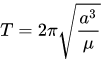# Astronomy help!

Astronomy help!
0

in Intermediate Algorithm Scripting: Map the Debris, i don’t understand
1)the math behind how i’m supposed to figure out the Orbital Period.
i also don’t get
2)what the altitude of an object has to do with an orbital period

if you post please the challenge link and the formula, the first question would be easier to answer

for the second, the altitude influence the gravitational force an object is subjected to (gravitational force has dependence on the distance), so it needs to be considered - that needs a bit of physics knowledge to understand

You will need to do some research for this challenge. There are many sites which show the formula needed to solve this challenge.

in short, i’m hopeless

thanks anyways

Nobody said that. Have you googled the terms in the problem?

iv’e only found a ‘How Stuff Works’ article on satellites, that doesn’t address the mathematics.

the wikipedia article in the challenge says this:which seems to be the most useful formula to use for this challenge, but i don’t know, because my knowledge of astronomy is very limited.
if the above formula were to be used, where `T` = time traveled (the number we are looking for? i don’t know) `a` = the orbit’s semi major axis, which i think is the provided 6367.4447 kilometers of earth. [which doesn’t make sense to me, since if we are calculating the radius of the orbit, which has space in between earth and the object, the radius of earth would give you a means of finding the orbit of an object taht is ON the surface, and not in space. which leaves me to believe that the altitude is the more important factor in determining the distance from the center, AND surface of earth, in which case, i have no idea how to calculate that number accurately] `pi` = 3.14 and `mew` = 398600.4418 km3s-2, as provided by the challenge.

but let’s assume i didn’t, what should i google?

can you provide the challenge link? you have not yet done that

You are on the right track actually. I would need to see the link to the challenge you are working on to give you more direct help, but the gap between the surface of the Earth and the orbit of the satellite is important.